Courses

# Test: Diode Rectifiers

## 10 Questions MCQ Test Topicwise Question Bank for Electronics Engineering | Test: Diode Rectifiers

Description
This mock test of Test: Diode Rectifiers for Electrical Engineering (EE) helps you for every Electrical Engineering (EE) entrance exam. This contains 10 Multiple Choice Questions for Electrical Engineering (EE) Test: Diode Rectifiers (mcq) to study with solutions a complete question bank. The solved questions answers in this Test: Diode Rectifiers quiz give you a good mix of easy questions and tough questions. Electrical Engineering (EE) students definitely take this Test: Diode Rectifiers exercise for a better result in the exam. You can find other Test: Diode Rectifiers extra questions, long questions & short questions for Electrical Engineering (EE) on EduRev as well by searching above.
QUESTION: 1

### The circuit shown below is initially relaxed. With switch closed at t = 0, the conduction time of diode is around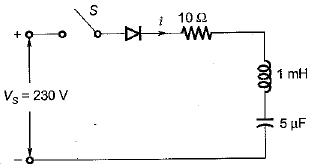Solution:

For the series RLC diode circuit, the current i(t) is given by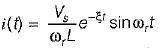Here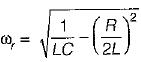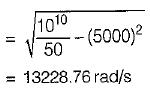The diode will stop conducting when ωr t1 = π.
∴ Conduction time of diode,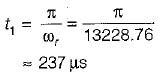QUESTION: 2

Solution:
QUESTION: 3

### A single-phase 230 V, 1 kW heater is connected across single-phase 230 V, 50 Hz supply through a diode as shown in figure. The power delivered to the heater element is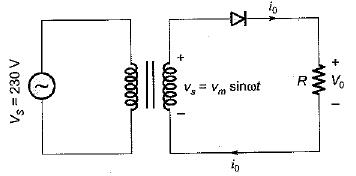Solution:

The rms value of output voltage is: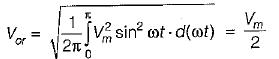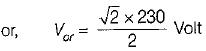Heater resistance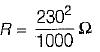∴ Power absorbed by heater element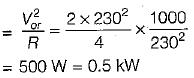QUESTION: 4

A single-phase full-bridge diode rectifier delivers a load current of 10 A, which is ripple free. The rms and average values of diode currents are respectively

Solution:

Given, I0 = 10A
The rms value of diode current,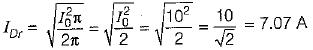The average value of diode current,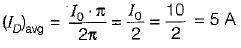QUESTION: 5

In the circuit shown below, the diode states at the extremely large negative value of the input voltage vi are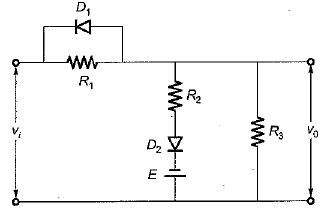Solution:

If vi is of very large negative value Ivi| > |E|, then diode D1 will be forward biased (ON) while diode D2 will be reverse biased (OFF).

QUESTION: 6

A single-phase full-bridge diode rectifier delivers a constant load current of 20 A. The rms and average values of source current are respectively

Solution:
QUESTION: 7

A diode whose internal resistance is 20 Q is to supply power to a 1 kΩ load from a 230 V (rms) source of supply as shown in figure below. The percentage regulation from no load to the given load is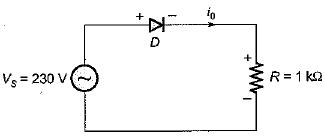Solution: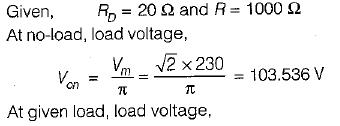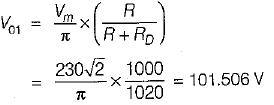∴ Voltage regulation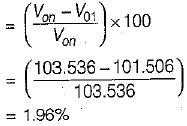QUESTION: 8

Assertion (A): A single-phase half-wave rectifier circuit is popularly known as one-pulse rectifier.
Reason (R) : A single-phase half-wave rectifier circuit is used to convert ac power to dc.

Solution:

A single-phase half-wave rectifier is popularly known as one-pulse converter because for one cycle of supply voltage, there is one half-cycle of output, or load voltage. Hence, both assertion and reason are true but reason is not the correct explanation of assertion.

QUESTION: 9

Assertion (A) : In 3-phase converters, the ripple frequency of the converter output voltage is higher than in single phase converter.
Reason (R) : The load current is mostly discontinuous in 3-phase converters.

Solution:

The load current is mostly continuous in 3-phase converters. Hence reason is a false statement.

QUESTION: 10

Assertion (A) : In a half-wave rectifier with R-L load and with freewheeling diode, the input Volt- Ampere demand is less.
Reason (R) : With freewheeling diode, the stored energy is in the load itself.

Solution: